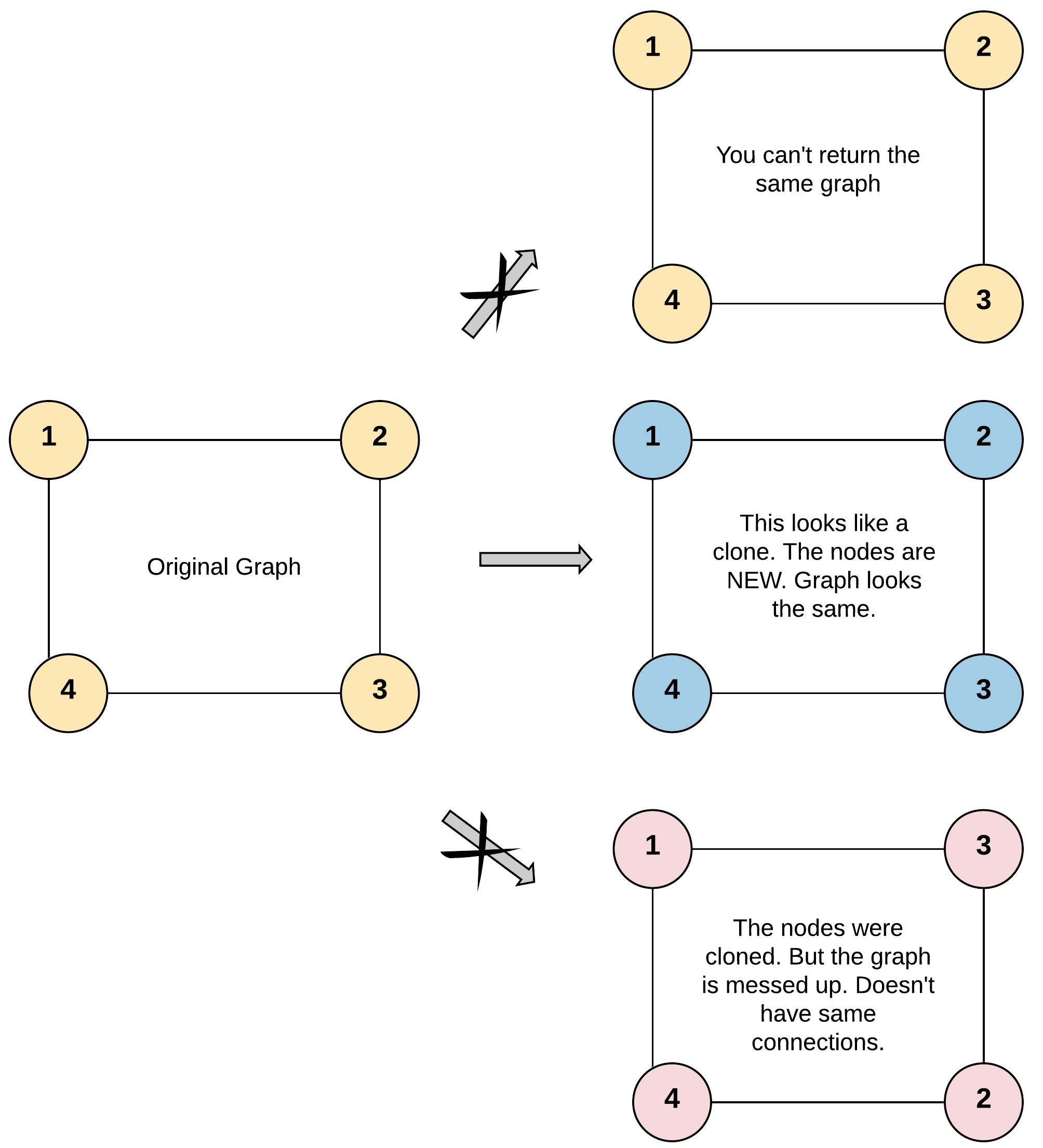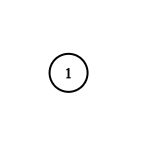## daily leetcode - clone-graph - !## 题目地址

https://leetcode.com/problems/clone-graph/

## 题目描述

Given a reference of a node in a connected undirected graph.

Return a deep copy (clone) of the graph.

Each node in the graph contains a val (int) and a list (List[Node]) of its neighbors.

``````class Node {
public int val;
public List<Node> neighbors;
}
``````

Test case format:

For simplicity sake, each node's value is the same as the node's index (1-indexed). For example, the first node with val = 1, the second node with val = 2, and so on. The graph is represented in the test case using an adjacency list.

Adjacency list is a collection of unordered lists used to represent a finite graph. Each list describes the set of neighbors of a node in the graph.

The given node will always be the first node with val = 1. You must return the copy of the given node as a reference to the cloned graph.

Example 1:``````Input: adjList = [[2,4],[1,3],[2,4],[1,3]]
Output: [[2,4],[1,3],[2,4],[1,3]]
Explanation: There are 4 nodes in the graph.
1st node (val = 1)'s neighbors are 2nd node (val = 2) and 4th node (val = 4).
2nd node (val = 2)'s neighbors are 1st node (val = 1) and 3rd node (val = 3).
3rd node (val = 3)'s neighbors are 2nd node (val = 2) and 4th node (val = 4).
4th node (val = 4)'s neighbors are 1st node (val = 1) and 3rd node (val = 3).
``````

Example 2:``````Input: adjList = [[]]
Output: [[]]
Explanation: Note that the input contains one empty list. The graph consists of only one node with val = 1 and it does not have any neighbors.
``````

Example 3:

``````Input: adjList = []
Output: []
Explanation: This an empty graph, it does not have any nodes.
``````

Example 4:``````Input: adjList = [,]
Output: [,]
``````

Constraints:

• 1 <= Node.val <= 100
• Node.val is unique for each node.
• Number of Nodes will not exceed 100.
• There is no repeated edges and no self-loops in the graph.
• The Graph is connected and all nodes can be visited starting from the given node.

## 代码

``````class Solution {
public:
Node* cloneGraph(Node* node) {
unordered_map<Node*, Node*> m;
return helper(node, m);
}
Node* helper(Node* node, unordered_map<Node*, Node*>& m) {
if (!node) return NULL;
if (m.count(node)) return m[node];
Node *clone = new Node(node->val);
m[node] = clone;
for (Node *neighbor : node->neighbors) {
clone->neighbors.push_back(helper(neighbor, m));
}
return clone;
}
};
``````

``````class Solution {
public:
Node* cloneGraph(Node* node) {
if (!node) return NULL;
unordered_map<Node*, Node*> m;
queue<Node*> q{{node}};
Node *clone = new Node(node->val);
m[node] = clone;
while (!q.empty()) {
Node *t = q.front(); q.pop();
for (Node *neighbor : t->neighbors) {
if (!m.count(neighbor)) {
m[neighbor] = new Node(neighbor->val);
q.push(neighbor);
}
m[t]->neighbors.push_back(m[neighbor]);
}
}
return clone;
}
};
``````评论
0 评论